Courses

# Morrison & Boyd Test: Some Basic Principles

## 25 Questions MCQ Test Chemistry for JEE | Morrison & Boyd Test: Some Basic Principles

Description
This mock test of Morrison & Boyd Test: Some Basic Principles for JEE helps you for every JEE entrance exam. This contains 25 Multiple Choice Questions for JEE Morrison & Boyd Test: Some Basic Principles (mcq) to study with solutions a complete question bank. The solved questions answers in this Morrison & Boyd Test: Some Basic Principles quiz give you a good mix of easy questions and tough questions. JEE students definitely take this Morrison & Boyd Test: Some Basic Principles exercise for a better result in the exam. You can find other Morrison & Boyd Test: Some Basic Principles extra questions, long questions & short questions for JEE on EduRev as well by searching above.
QUESTION: 1

### Write the state of hybridisation of carbon in H2C=O.

Solution:

C in H2C=O is sp2 hybridised and geometry is planar.

QUESTION: 2

### What is the correct order of decreasing stability of the following cations.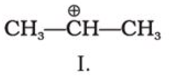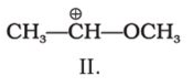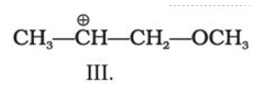Solution:

Stability of carbocation increases with the dispersal of positive charge by the donation of eletron density through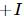effect The groups showingeffect are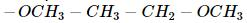thus the correct stability order of the given carbocations is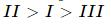QUESTION: 3

### C4H10O represents methoxypropane (CH3OC3H7) and ethoxyethane (C2H5O C2H5)

Solution:

rearrangement of carbon atoms around the functional group is known as metamerism.

QUESTION: 4

Electrophilic addition reactions proceed in two steps. The first step involves the addition of an electrophile. Name the type of intermediate formed in the first step of the following addition reaction.

H3C−−−HC = CH2+ H+→?

Solution:

A secondary carbocation is formed.

QUESTION: 5

During estimation of nitrogen present in an organic compound by Kjeldahl’s method, the ammonia evolved from 0.5 g of the compound, neutralized 10 mL of 1 M H2SO4.Find out the percentage of nitrogen in the compound.

Solution:

moles of NH3= moles of N= 2 × 10 ×1/1000 = 0.02 moles Mass of N = 0.02 × 14 % = 0.02 x 14 × 100/0.5 = 56%

QUESTION: 6

The IUPAC name for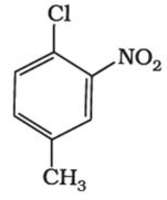Solution:

1-Chloro-4-methyl-2-nitrobenzene is the iupac name of the given compound.

QUESTION: 7

What is the type of hybridisation of each carbon in the compound (CH3)2CO?

Solution:

C in CH3 is sp3 and C of C=O bond is sp2.

QUESTION: 8

The organic compound given below is one of the four options given below. Choose the most appropriate one.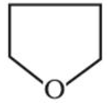Solution:

this is aliphatic heterocyclic compound.

QUESTION: 9

In general, in the molecules containing multiple bonds,_____ provide the most reactive centres. Choose the most appropriate one.

Solution:

pie bonds are the most reactive centers.

QUESTION: 10

Which of the following is the correct IUPAC name?

Solution:

While writing IUPAC name, the alkyl groups are written in alphabetical order. Thus lower locant 3 is assigned to ethyl. Prefix, di, tri, and tetra are not included in alphabetical order.QUESTION: 11

In a π (pi) bond formation, is necessary for one of the below criteria. Choose the appropriate one.

Solution:

parallel orientataion of p orbitals are necessary for proper overlapping and formation of pie bond.

QUESTION: 12

Alkyl radical (Methyl, Ethyl, Isopropyl, Tert-butyl free radical) stability in the increasing order is:

Solution:

tertiary radical is more than secondary and primary.

QUESTION: 13

The order of decreasing priority for some functional groups in the naming of an organic compound is:

Solution:

COOR (R=alkyl group), -COCl, -CONH2, -CN,-HC=O, >C=O, -OH, −NH2 is the decreasing order of priority

QUESTION: 14

Covalent bond can undergo fission in two different ways. The correct representation involving a heterolytic fission of CH3−−−Br is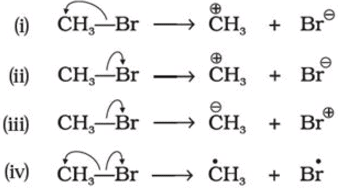Solution:

arrows are shown in the direction of movement of electrons.

QUESTION: 15

When inductive and electromeric effects operate in opposite directions, what happens?

Solution:

When inductive and electromeric effects operate in opposite directions then electomeric effect predominates

QUESTION: 16

In general, in the molecules containing multiple bonds,_____ provide the most reactive centres. Choose the most appropriate one.

Solution:

pie bonds are the most reactive centers.

QUESTION: 17

The nitrogen containing organic compound, when heated with copper oxide in an atmosphere of carbon dioxide, yields:

Solution:

The nitrogen containing organic compound, when heated with copper oxide in an atmosphere of carbon dioxide, yields free nitrogen

QUESTION: 18

Which of the following statement is not correct for a nucleophile?

Solution:

Nucleophile is not Lewis acid. Lewis acids are those substances which accept lone pairs. However, nucleophiles itself are lone pair donors.

QUESTION: 19

The addition of HCl to an alkene proceeds in two steps. The first step is the attack of H+ ion to portion which can be shown as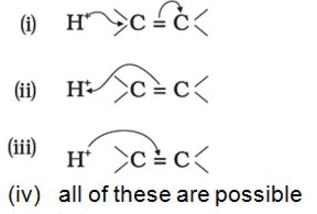Solution:

It is the correct representation since double bond has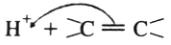high electron density, it provides electron pair for attack on H+. A carbocation results.

QUESTION: 20

What does the following diagram showing Lewis structures depict?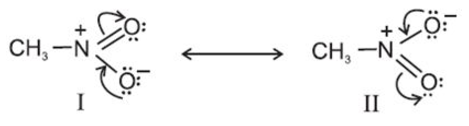Solution:

they are resonating structures of nitromethane

QUESTION: 21

Which of the following compounds contain all the carbon atoms in the same hybridisation state?

Solution:

In A all Carbon atoms are sp hybridized

QUESTION: 22

A known mass of an organic compound is heated with fuming nitric acid in the presence of silver nitrate is:

Solution:

This is known as carius method.

QUESTION: 23

For testing halogens in an organic compound with AgNO3 solution, sodium extract (Lassaigne’s test) is acidified with dilute HNO3.What will happen if a student acidifies the extract with dilute H2SO4 in place of dilute HNO3?

Solution:

white precipitate of Ag2SO4 is formed.

QUESTION: 24

Nucleophile is a species that should have:

Solution:

nucleophile should have negative charge or pair of electron to donate.

QUESTION: 25

Delocalisation of σ electrons of C -H bond of an alkyl group directly attached to an atom of unsaturated system or to an atom with an unshared p orbital is called :

Solution:

Delocalisation of σ electrons of C -H bond of an alkyl group directly attached to an atom of unsaturated system or to an atom with an unshared p orbital is known as Hyperconjugation